Introduction to Tableau and Data Visualization
)}

# Aggregation and Granularity

‍If one was to remove the categories pill, you would only see a single bar. Why?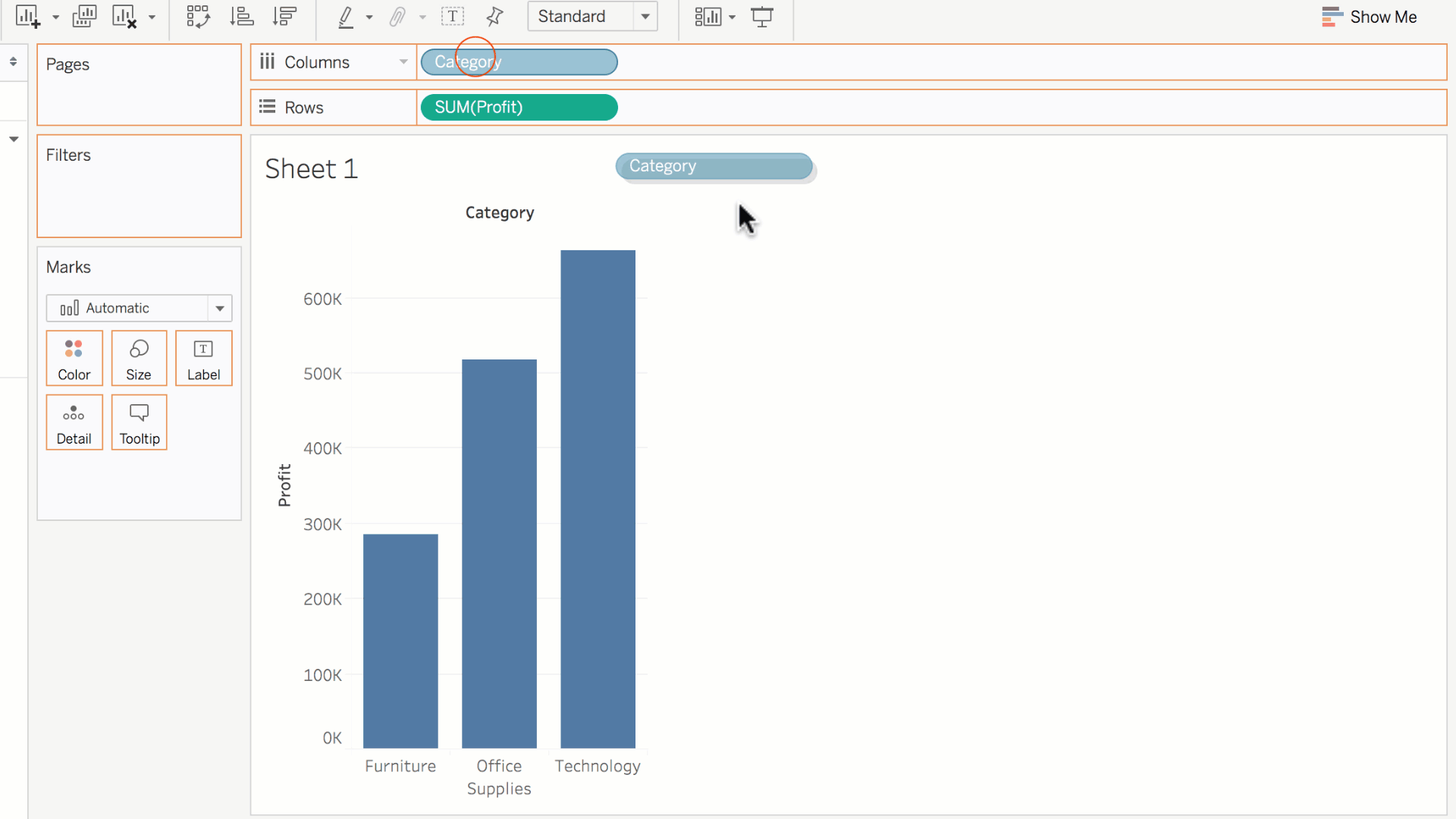This goes back to the subject of **granularity. **Recall:

In Tableau, we use dimensions to specify the granularity of a measure's calculation.

In this case, there is **no dimension specified for the calculation, **so the calculation becomes less granular. Tableau simply sums up the profit for all the orders in the dataset into a single value, and displays it as a single bar.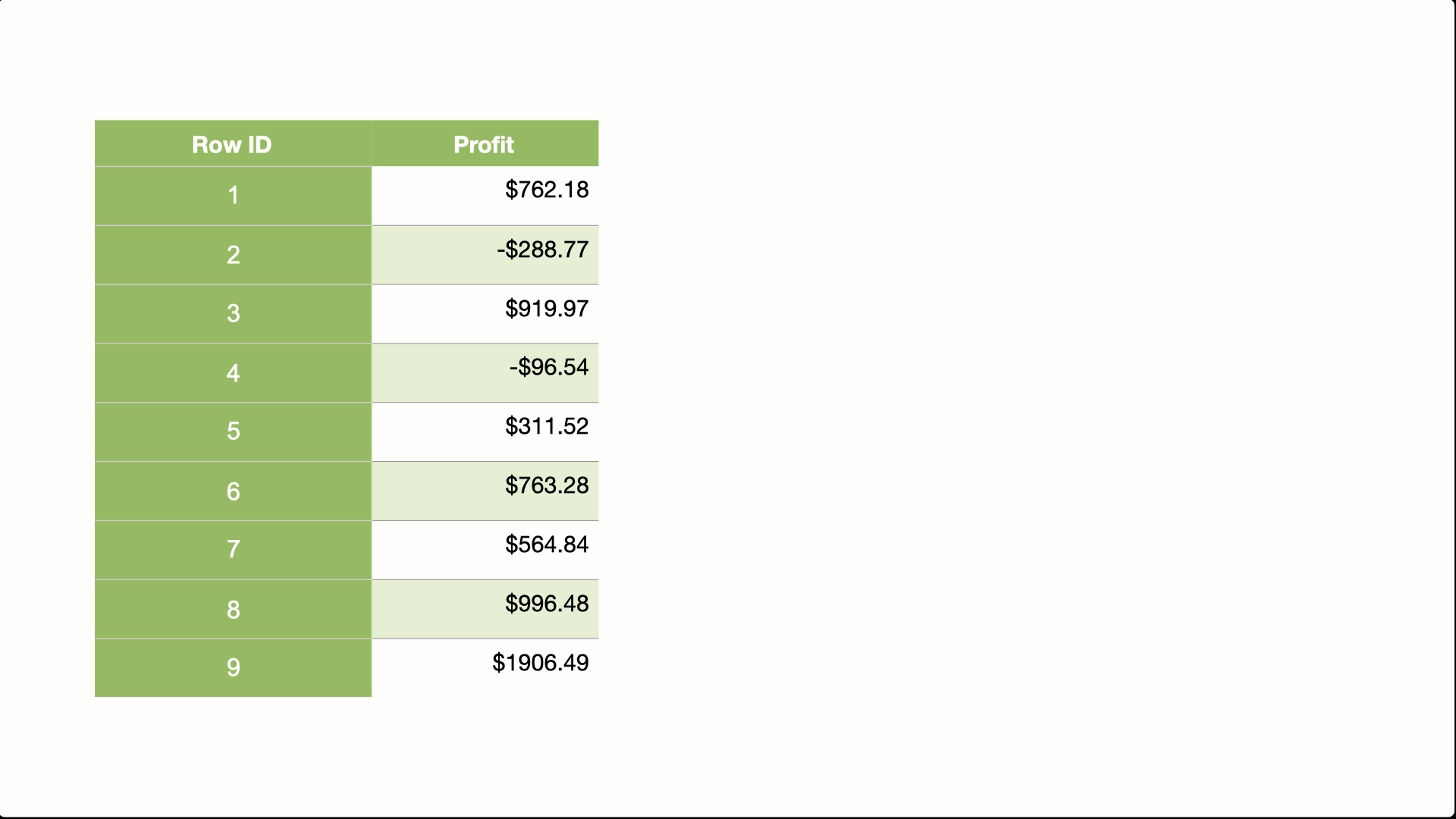However, by dragging Categories back into Columns, we increase the granularity of the calculation.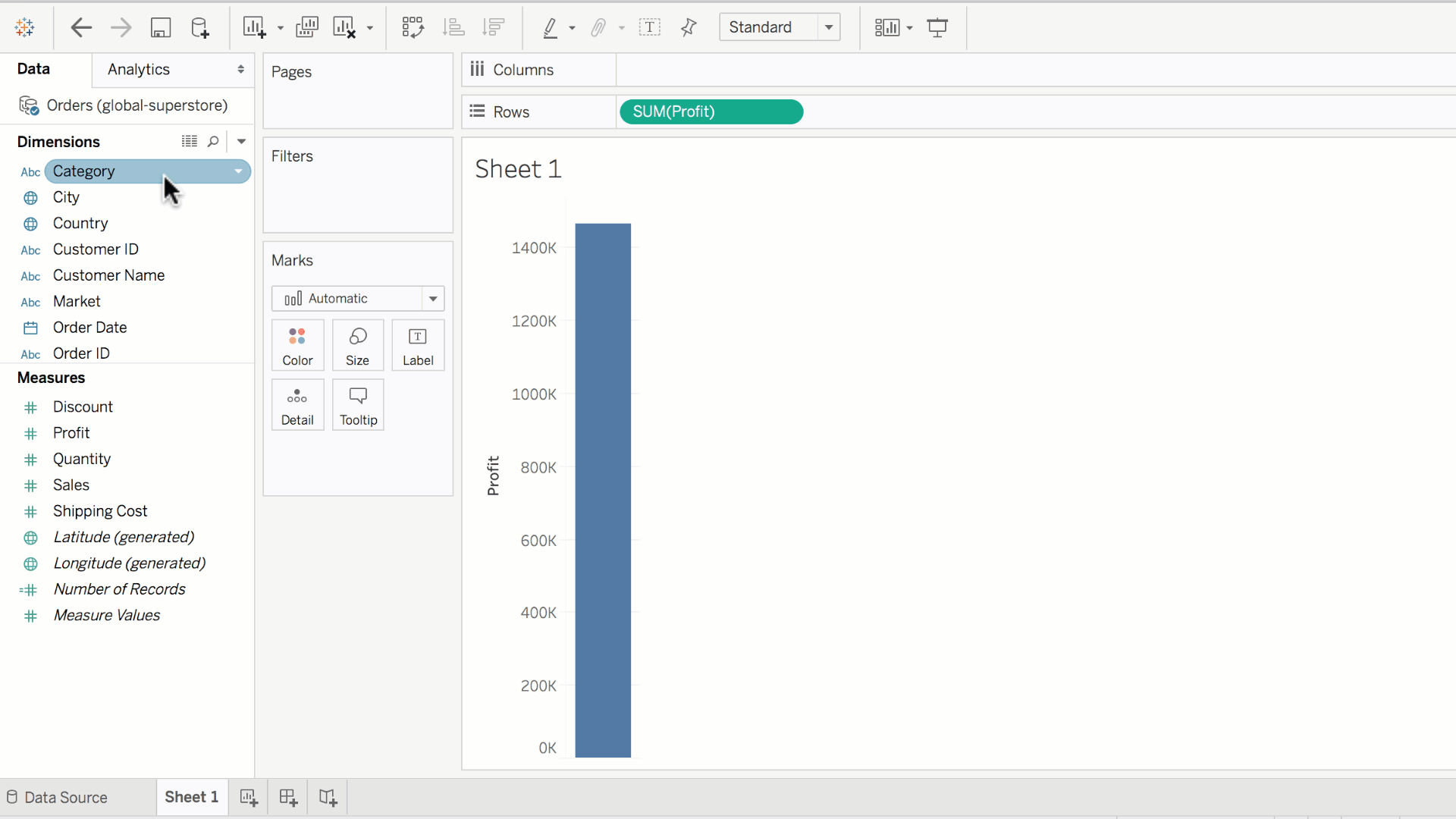This means that we're not just aggregating over all the records, but we're aggregating the profit sum over each category, displaying each calculation as a bar.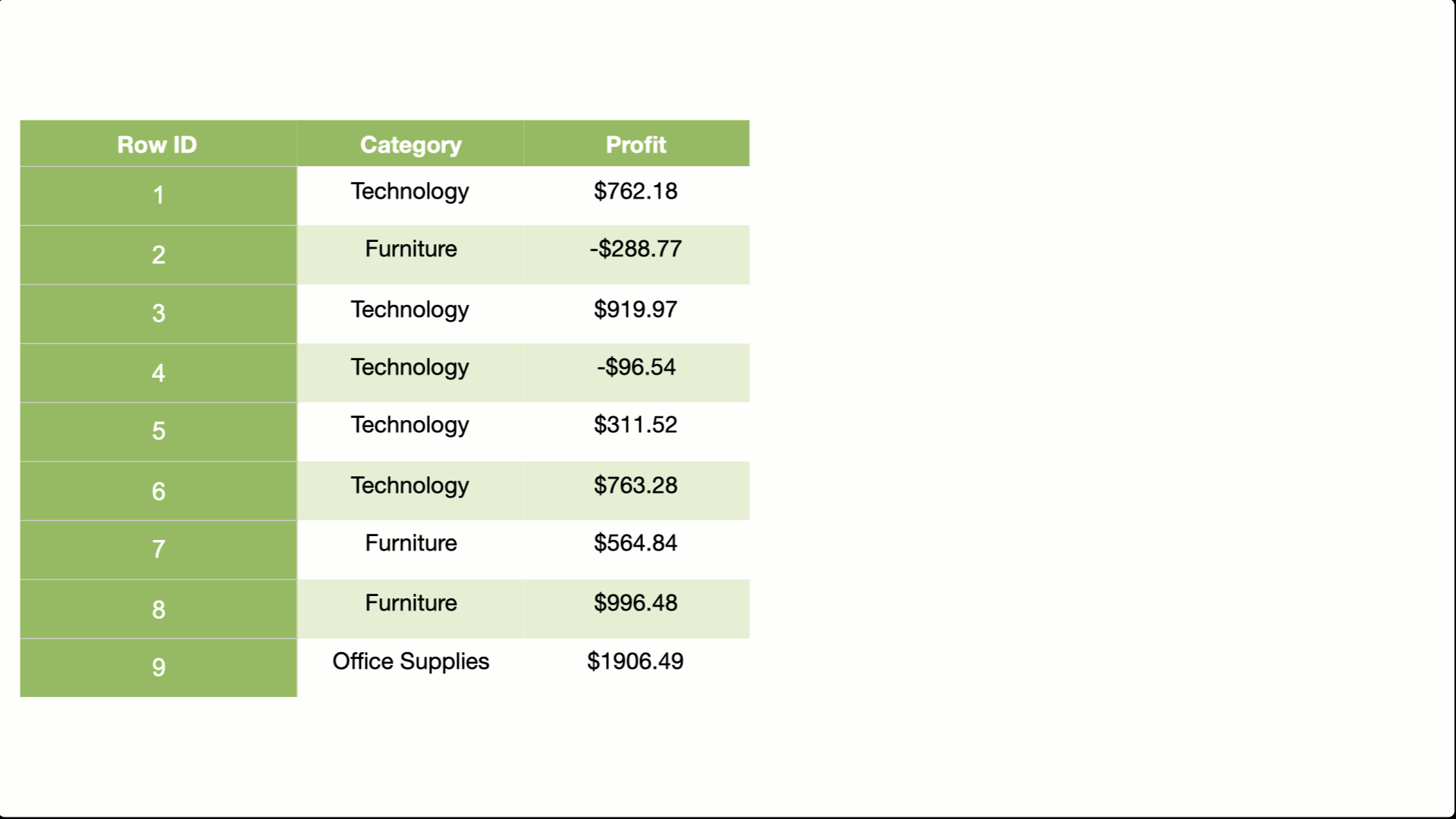Say we wanted to know, why is furniture making the least profit? We can increase the granularity and aggregate over the subcategories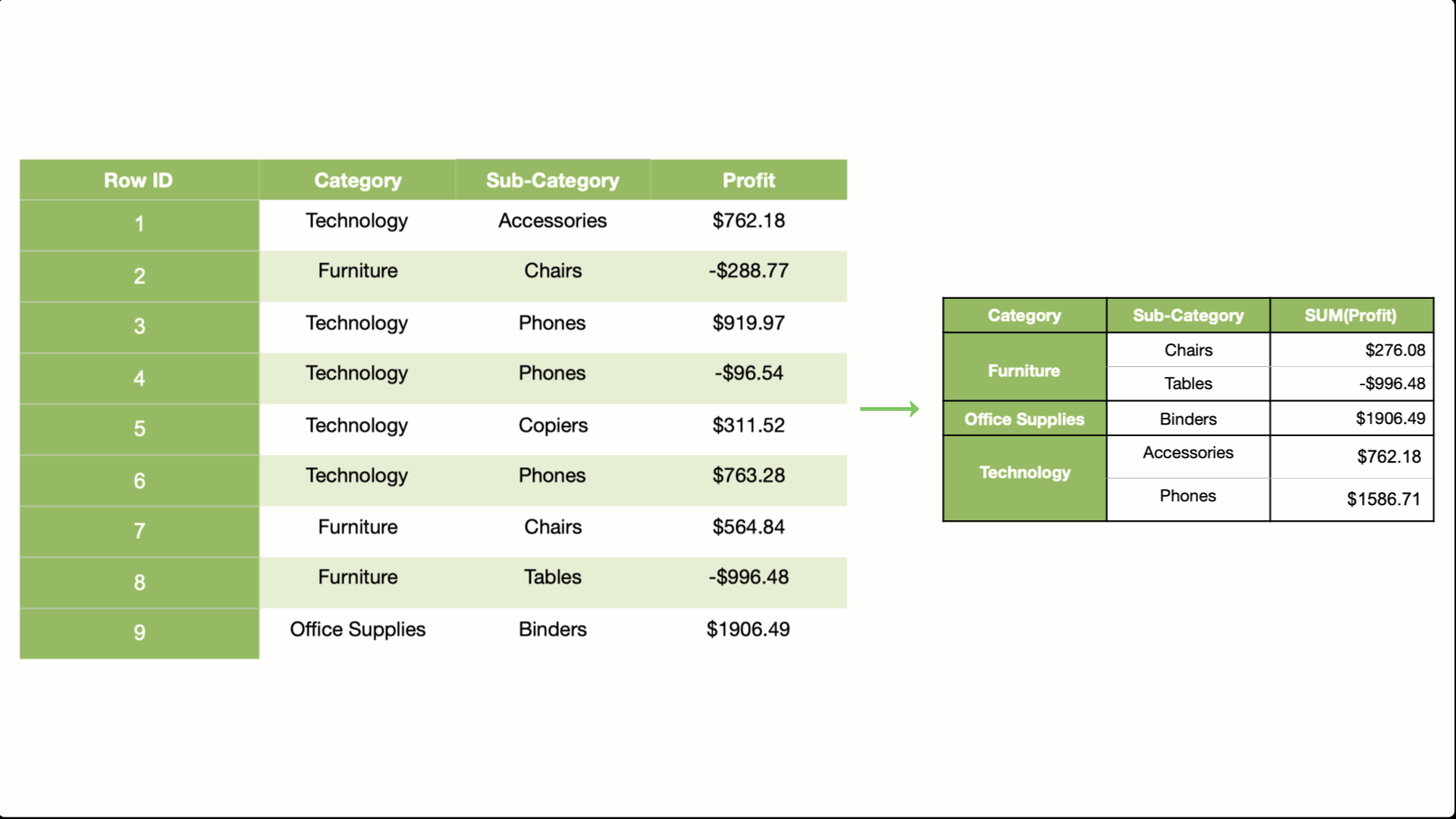You can do this in tableau by simply adding sub-category to the column shelf.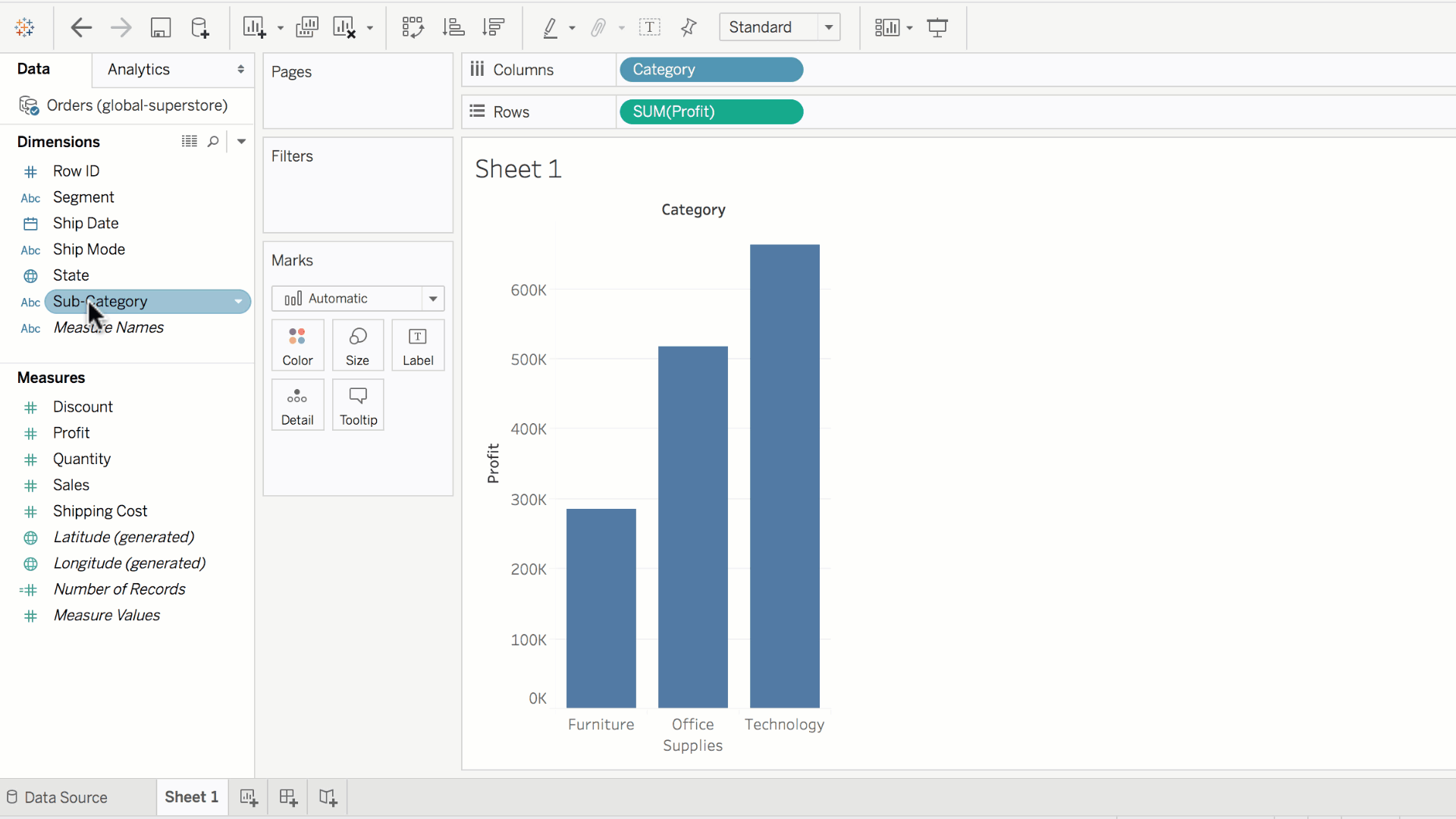We can see that the tables sub-category within the furniture category is actually losing money, driving the overall furniture profits down.

Let's remove the sub-categories field for now, and revert the calculation to aggregate over only categories.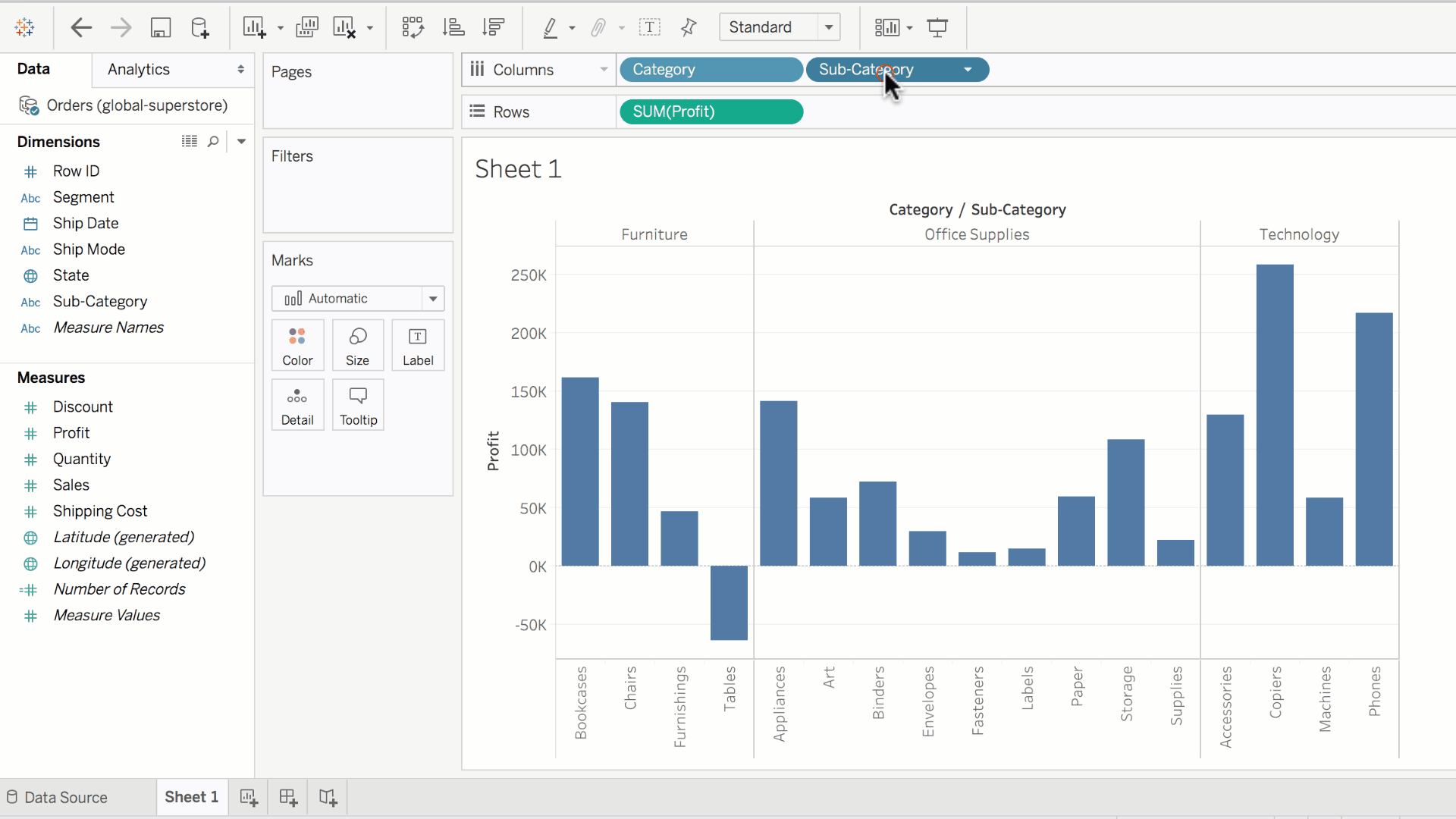We will now increase the granularity to aggregate the profits over each category, at each year. As there are 4 years, and three categories, this will result in 12 profit sums. Each of these sums can be visualized as a bar, in a bar plot.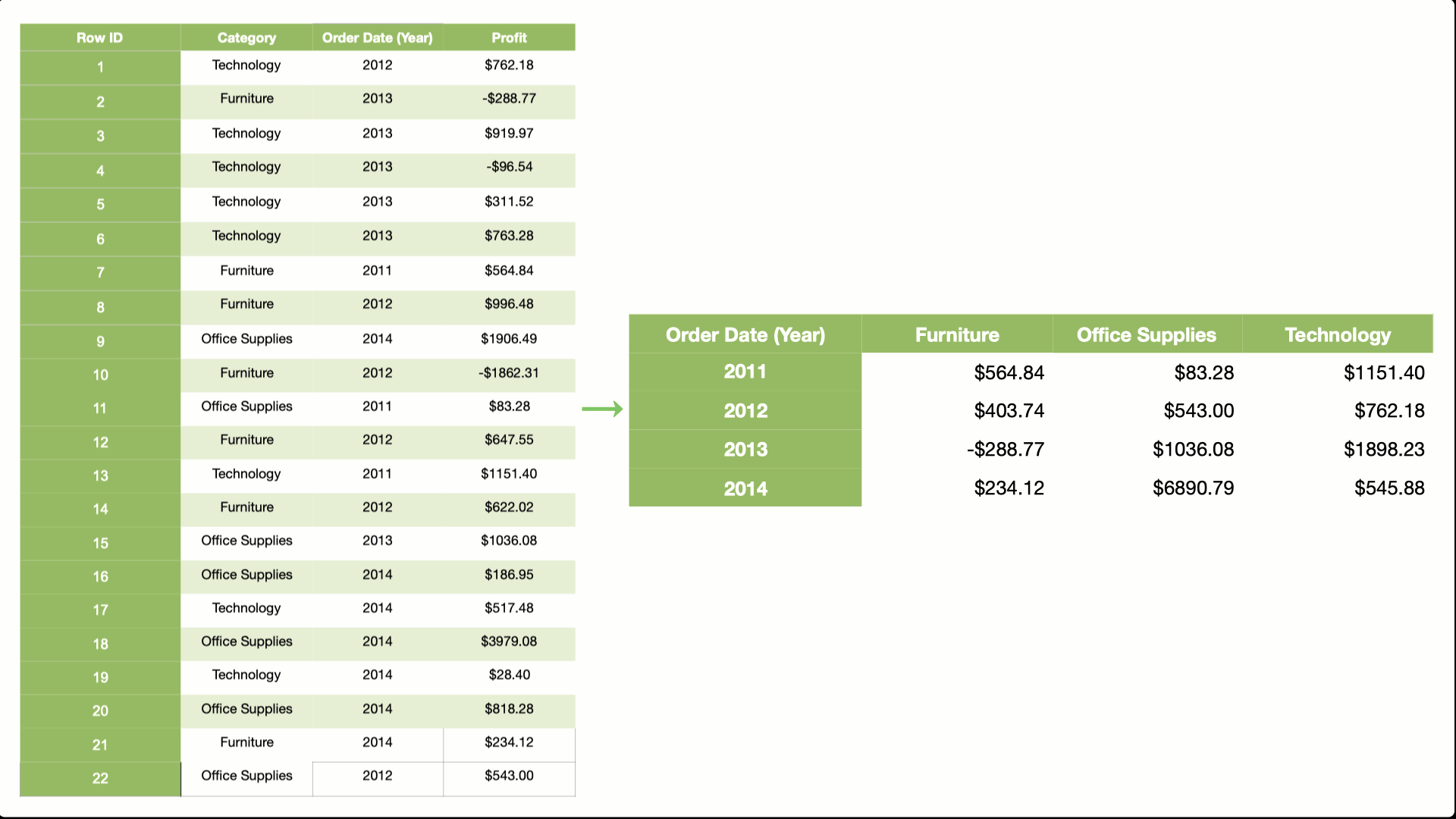This is done in Tableau by dragging the Order Date field into the columns shelf. Dates are dimensions by definition, since they categorize our data in terms of year, month or day. By dragging Order Date to columns, the granularity is increased to calculate the sum of profit for each category, at each year.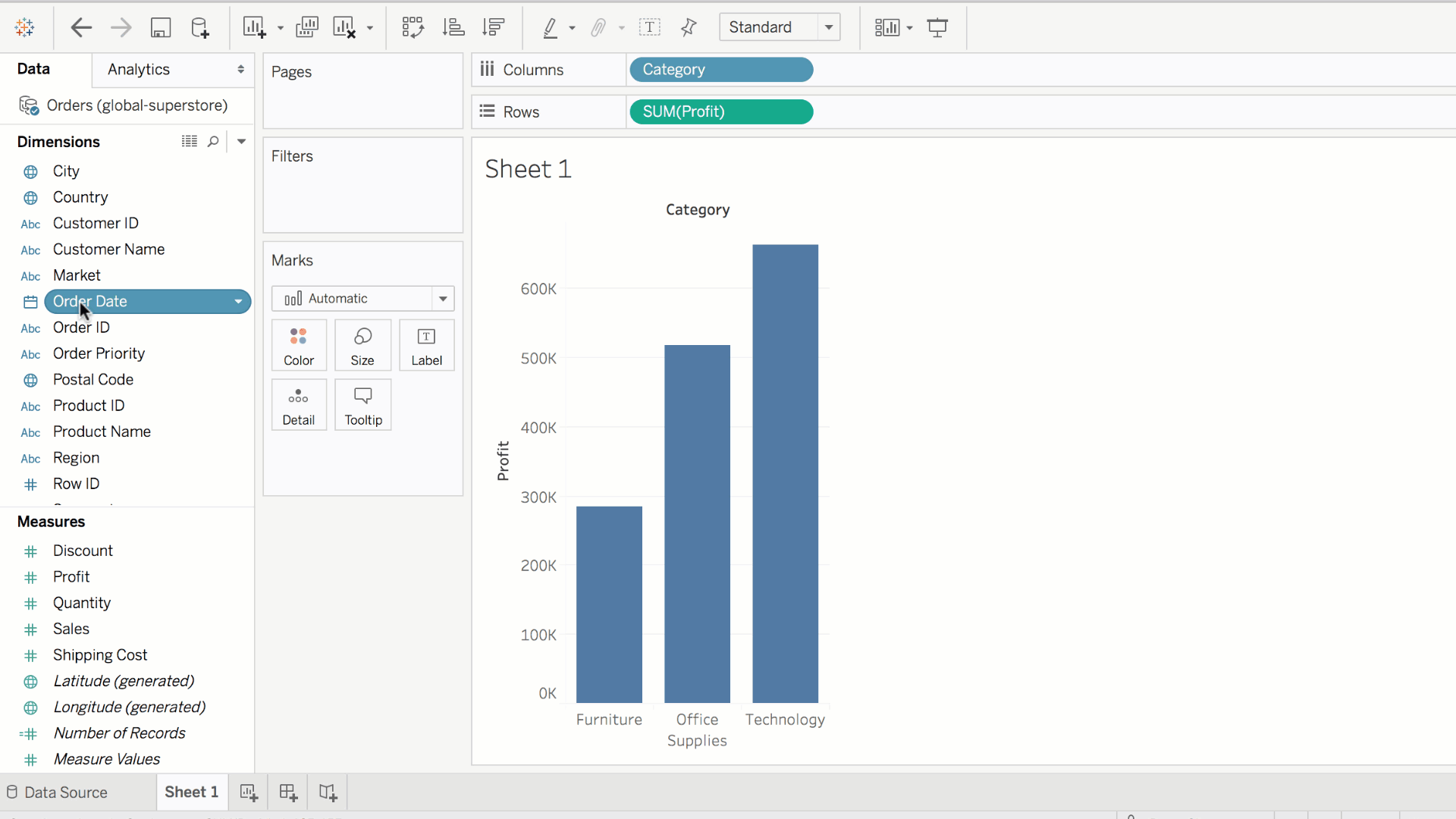Tableau's default for displaying time data is a line graph. We can also visualize this in a bar plot.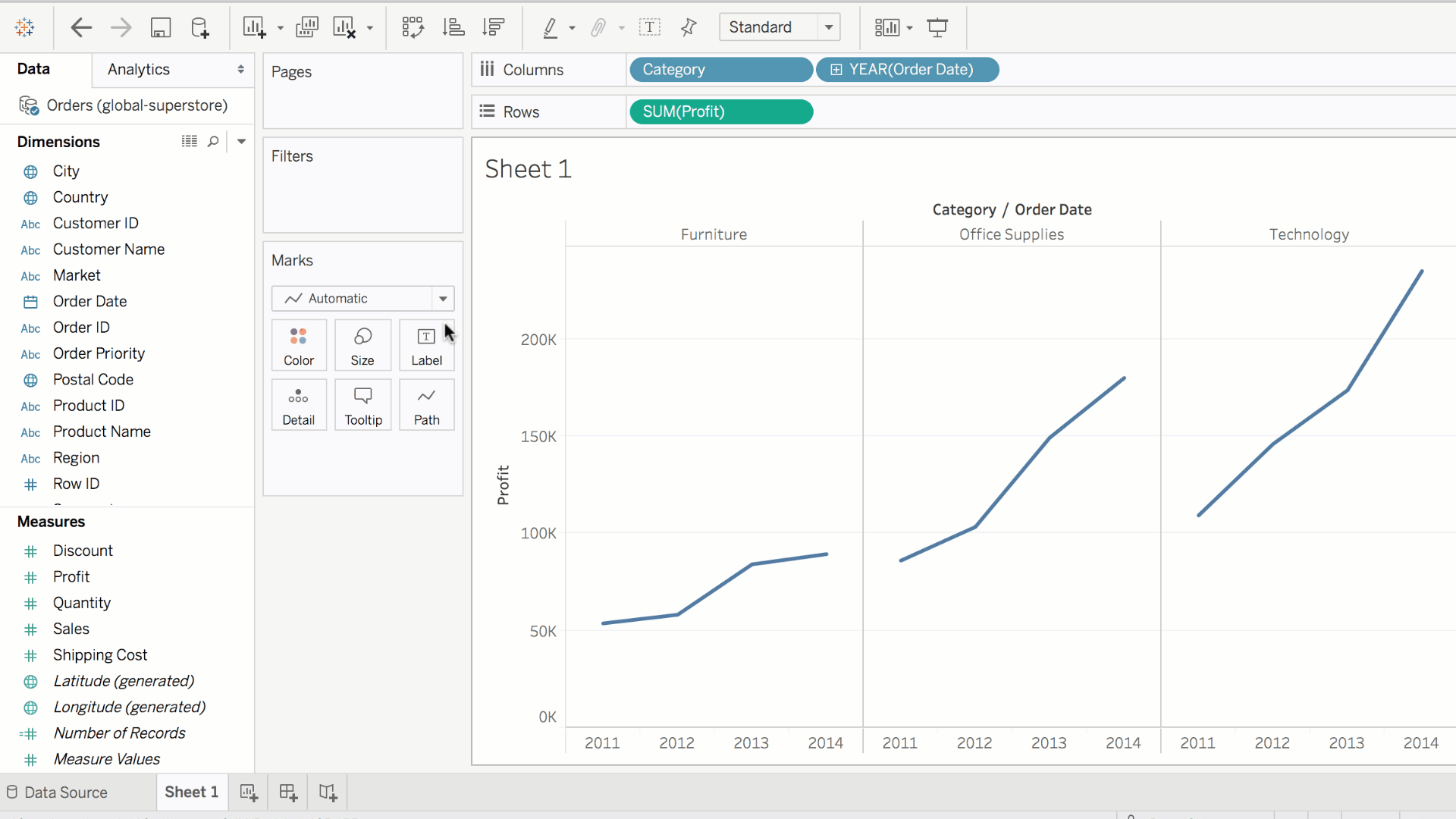Easy right? All we did was use dimensions to specify the granularity of the calculation.

Controlling the scope of a calculation based on a discrete dimension is a very powerful way of visualizing data, and it's fundamental to all calculations that are performed in Tableau.

Feedback Summary
5.0
2 students
5

100%
4

0%
3

0%
2

0%
1

0%
Written Reviews
There are no written reviews yet.